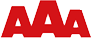## Body Mass Index calculator

Calculating your BMI is a popular and easy way to tell if your weight is putting your health at risk. Our example spreadsheet calculates your BMI after you’ve entered your weight and height.

The higher the BMI, the greater the risk of developing health problems. The World Health Organization (WHO) regard a BMI of less than 18.5 as underweight and a BMI of 25 or more as overweight.

Test this calculator in other formats:

## Description

Calculating your BMI is a popular and easy way to tell if your weight is putting your health at risk.

The higher the BMI, the greater the risk of developing health problems. The World Health Organization (WHO) regard a BMI of less than 18.5 as underweight and a BMI of 25 or more as overweight.

BMI is not undisputed. There are several equally simple ways to check your weight, e.g. by measuring your waist. Studies suggest that health risks begin to increase when a woman’s waist reaches 31.5 inches (80 cm). For men, risk starts to climb at 37 inches (94 cm).

BMI is calculated using the following formulas:

Units Formula and calculation
Kilograms and meters Formula: weight (kg) / [height (m)]2
With the metric system, the formula for BMI is weight in kilograms divided by height in meters squared. Since height is commonly measured in centimeters, divide height in centimeters by 100 to obtain height in meters.
Example: Weight = 68 kg, Height = 165 cm (1.65 m)
Calculation: 68 ÷ (1.65 x 1.65) = 24.98
Pounds and inches Formula: weight (lb) / [height (in)]2 x 703
Calculate BMI by dividing weight in pounds (lbs) by height in inches (in) squared and multiplying by a conversion factor of 703.
Example: Weight = 150 lbs, Height = 5’5” (65″)
Calculation: 150 ÷ (65 x 65) x 703 = 24.96

Open the html version of the BMI Body Mass Index calculator.

Return to the list of examples of what SpreadsheetConverter can do.

## Try this Excel add-in now!Click on Download to install and test this Excel add-in for Windows.